Monday, September 27, 2021
Home > geometry > SSC CGL Geometry Shaded Area Set 1

# SSC CGL Geometry Shaded Area Set 1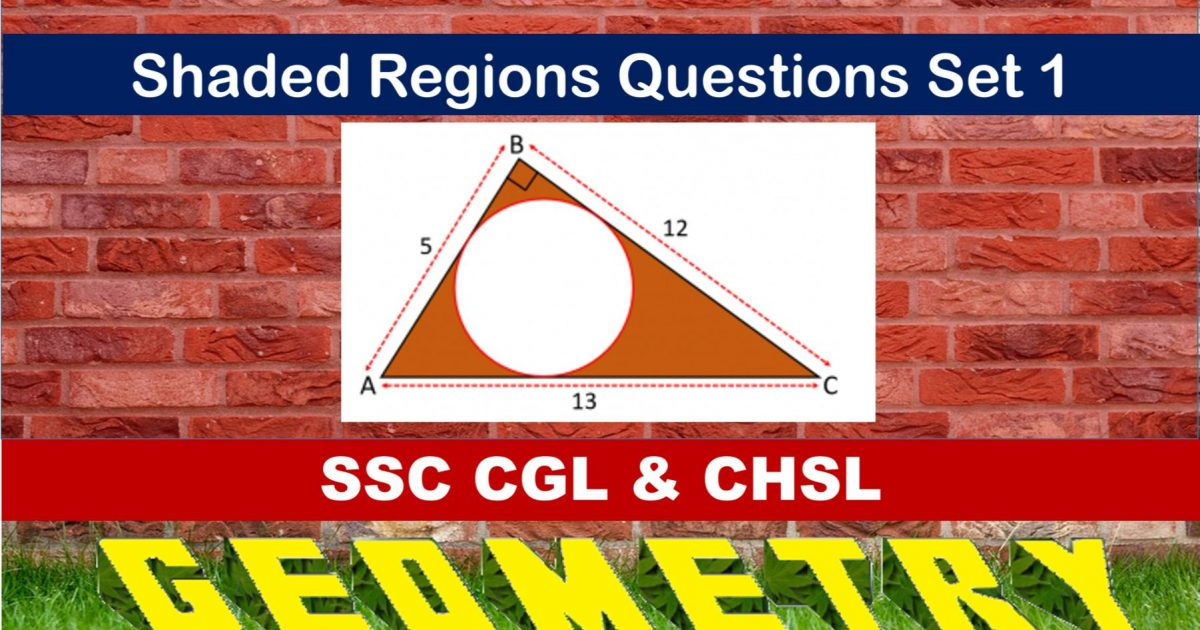SSC CGL CHSL Shared Area & Perimeter
Shaded Area & Perimeter Questions Sets
Set 1Set 2Set 3

SSC CGL Geometry Shaded Area Set 1 : Ques No 1

In the figure below, find the shaded area.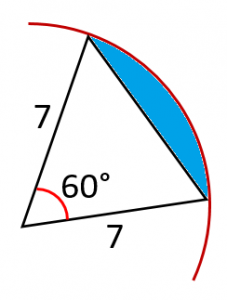Options:

A. 2(11/3 – 7√3/4)

B. 7(11/3 – 7√3/4)

C. 3(1/3 – 5√3/4)

D. 4(11/3 – 5√3/4)

Solution:SSC CGL Geometry Shaded Area Set 1 : Ques No 2

In the figure below, find the area of shaded region.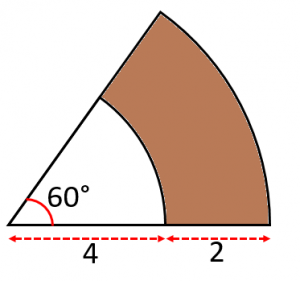Options:

A. Pi/3

B. 5Pi/3

C. 7Pi/3

D. 10Pi/3

Solution:SSC CGL Geometry Shaded Area Set 1 : Ques No 3

In the figure below, a circle is inscribed in a semi-circle, then find the area of shaded region.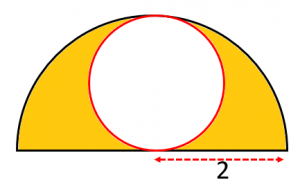Options:

A. 2Pi

B. 3Pi

C. Pi

D. 4Pi

Solution: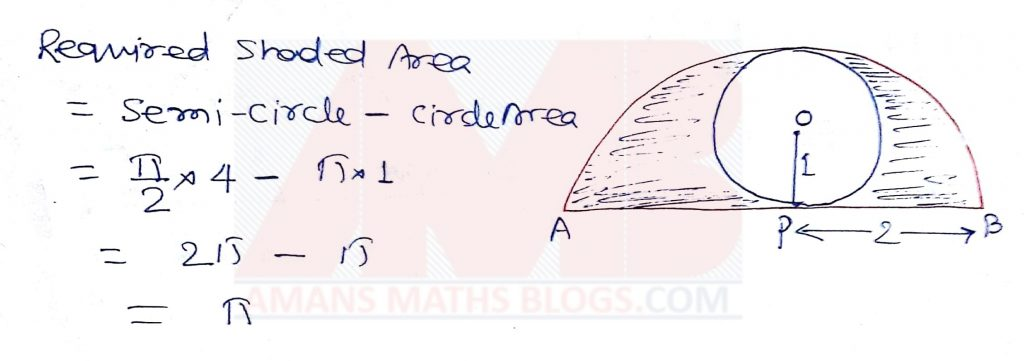SSC CGL Geometry Shaded Area Set 1 : Ques No 4

In the figure below, two sector of circle with radius 6 cm are drawn in an equilateral triangle, then find the shaded area.Options:

A. 12(3√3 – Pi)

B. 6(3√3 – 2Pi)

C. 2(2√3 – Pi)

D. 5(3√3 – 2Pi)

Solution: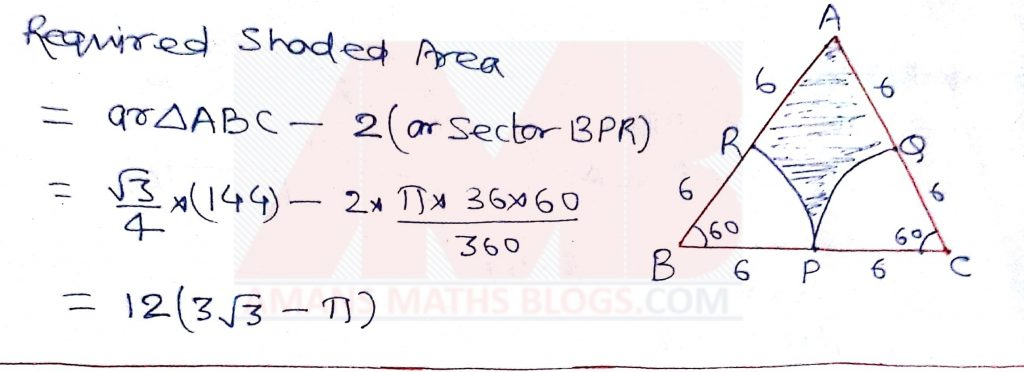SSC CGL Geometry Shaded Area Set 1 : Ques No 5

In the figure below, a semi-circle is inside a quarter circle of radius 6 units, then find the shaded area.Options:

A. 9Pi

B. 9Pi/2

C. 9Pi/4

D. 9Pi/8

Solution:SSC CGL Geometry Shaded Area Set 1 : Ques No 6

In the figure below, three sector of circles are drawn with center as the vertex of an equilateral triangle, then find the shaded area.Options:

A. (2√3 – Pi)

B. 2(2√3 – Pi)

C. 3(2√3 – Pi)

D. 4(2√3 – Pi)

Solution: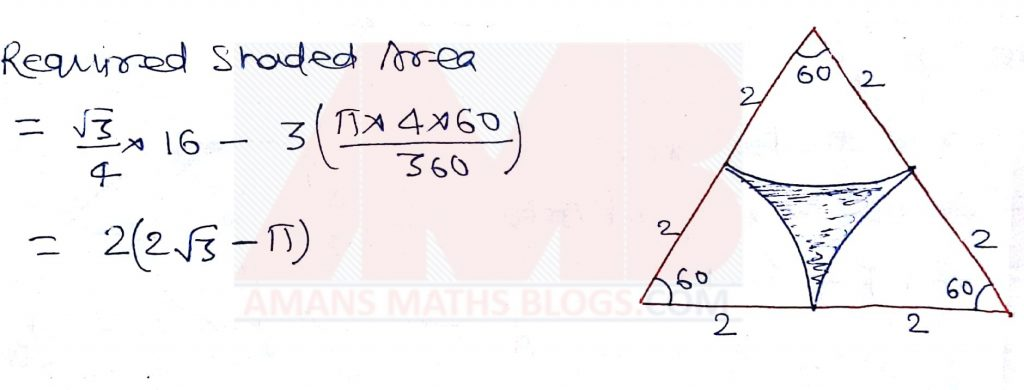SSC CGL Geometry Shaded Area Set 1 : Ques No 7

In the figure below, a square is of side 2 units, then find the shaded area.Options:

A. 2(Pi – 2)

B. 3(Pi + 2)

C. 4(Pi – 2)

D. 5(Pi + 2)

Solution: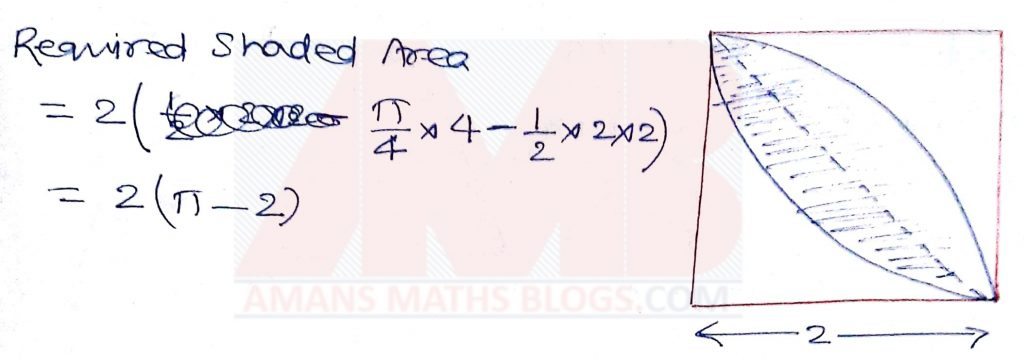SSC CGL Geometry Shaded Area Set 1 : Ques No 8

In the figure below, a circle is  inscribed in an equilateral triangle with side  6 units, then find the shaded area.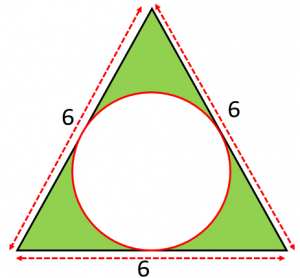Options:

A. 3(4√3 – 2Pi)

B. 2(√3 – Pi)

C. 3(3√3 – Pi)

D. 3(√3 – 3Pi)

Solution: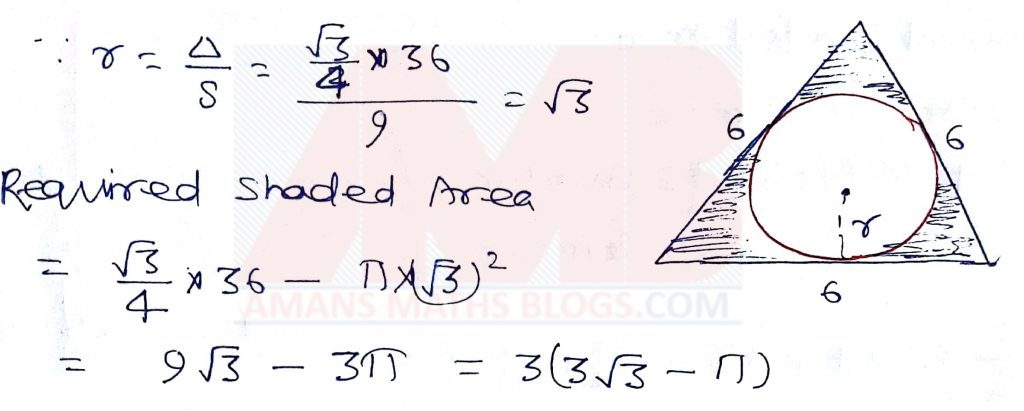SSC CGL Geometry Shaded Area Set 1 : Ques No 9

In the figure below, a circle is  inscribed in a right triangle with sides  5, 12 and 13 units, then find the shaded area.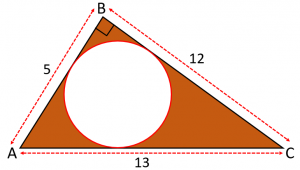Options:

A. 2(15 – 2Pi)

B. 2(15 – 3Pi)

C. 2(15 – 4Pi)

D. 2(15 – 5Pi)

Solution:SSC CGL Geometry Shaded Area Set 1 : Ques No 10

In the figure below, ABCD is a trapezium with AB || CD, then find the ratio of shaded area to the trapezium area, (means ar(BCD) : ar(ABCD))Options:

A. 1 : 3

B. 2 : 5

C. 4 : 7

D. 3 : 8

Solution:SSC CGL Geometry Shaded Area Set 1 : Ques No 11

Points B, D, and J are midpoints of the sides of right triangle ACG. Points K, E, I are midpoints of the sides of triangle JDG, etc. If the dividing and shading process is done 100 times (the first three are shown) and AC = CG = 6, then the total area of the shaded triangles is nearest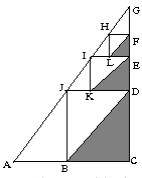Options:

A. 6

B. 7

C. 8

D. 9

E. 10

SSC CGL Geometry Shaded Area Set 1 : Ques No 12

Figure ABCD is a square. Inside this square three smaller squares are drawn with side lengths as labeled. The area of the shaded L-shaped region isOptions:

A. 7

B. 10

C. 12.5

D. 14

E. 15